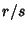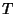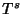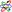## Poincaré-Birkhoff Fixed Point Theorem

For the rational curve of an unperturbed system with Rotation Numberunder a map(for which every point is a Fixed Point of), only an even number of Fixed Points(, 2, ...) will remain under perturbation. These Fixed Points are alternately stable (Elliptic) and unstable (Hyperbolic). Around each elliptic fixed point there is a simultaneous application of the Poincaré-Birkhoff fixed point theorem and the KAM Theorem, which leads to a self-similar structure on all scales.

The original formulation was: Given a Conformal One-to-One transformation from an Annulus to itself that advances points on the outer edge positively and on the inner edge negatively, then there are at least two fixed points.

It was conjectured by Poincaré from a consideration of the three-body problemin celestial mechanicsand proved by Birkhoff.